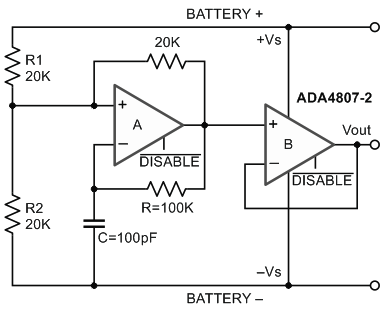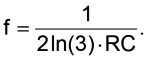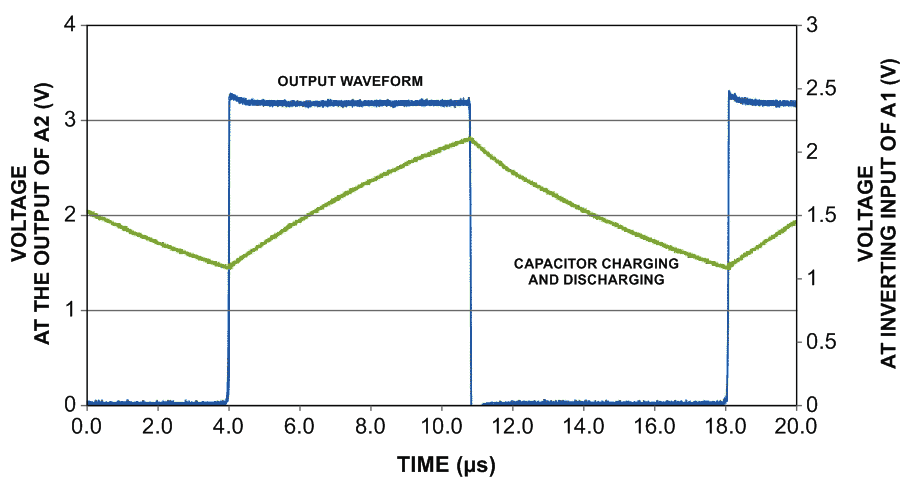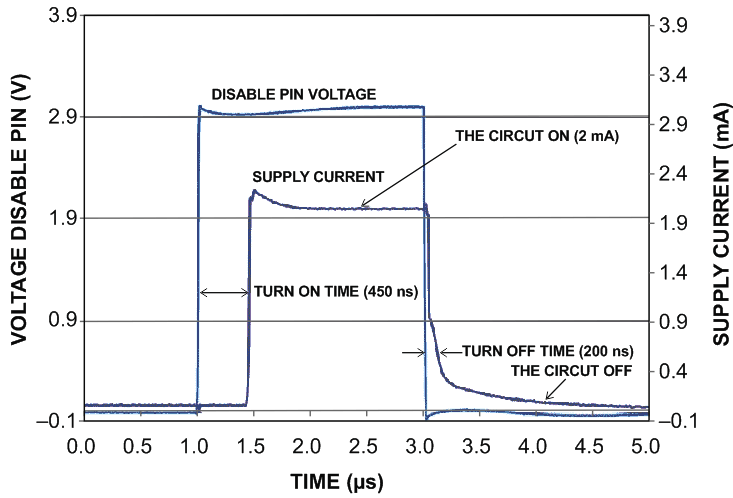# Low-Power, Battery Powered Square Wave Oscillator with Hibernation Mode

By Chau Tran, Analog Devices

EDN Europe

In many audio, automotive and instrumentation applications, there are requirements for a low cost but highly stable and accurate square wave generator with a respectable level of output current drive. As we have often heard, there’s always a demand for a low cost solution for a high performance application. The circuit shown in Figure 1 addresses this demand by using only a low-cost dual op-amp with an optional shut-down function, and a few passive components.Figure 1. The square wave oscillator.

The circuit of Figure 1 employs an ADA4807-2, which is a dual, low power, low noise, rail to rail voltage feedback amplifier. The first op-amp, A, is the main square waveform generator, and the second one, B, is just the driver. This buffer can typically source or sink a linear output current of ±40 mA. Generally, op-amp A acts like a comparator; there is a positive feedback loop in the presence of a slow negative feedback loop triggered by the RC circuit, which causes the circuit to oscillate automatically. When the capacitor reaches each threshold, the charging source is switched from the negative power supply to the positive power supply and vice versa.

The system is in unstable equilibrium if both the inputs and outputs of the comparator are at exactly zero volts. However, any noise will bring the output of the comparator above or below zero and the positive feedback in the comparator forces the output of the comparator to be at the rail until the next cycle.

Between the inverting input and the output of the comparator is a RC circuit. Because of this, the inverting input of the comparator asymptotically approaches the comparator output voltage with a time constant RC.

This time constant RC determines the frequency of the oscillation:The duty cycle of the signal can be adjusted by changing the ratio of resistors R1 to R2. In this circuit, those two resistors are equal; therefore, the output is symmetrical.

The output of the driver swings as far as 40 mV from the +/- rail with a linear output current source of 50 mA or sink of 40 mA (typically).Figure 2. The output waveform of the low cost square wave oscillator.

The oscillator can be switched on and off via DISABLE pins. This “hibernation” functionality of the disable circuit is a useful power reduction option. When the circuit is not in use, one can turn it off by pulling down the DISABLE pins to less than 1.1 V. This will automatically reduce the supply current of the two op-amps to a negligible level within a 200 nsec time frame. The remaining system current is mostly from the resistor divider R1 and R2. Once the device goes into hibernation mode the operating current of the battery is reduced from 2 mA to 80 μA. The output enters a high impedance state but it takes less than 500 nsec for it to wake up and start running.Figure 3. The DISABLE functionality turns the circuit on and off quickly.

The dual op amp, shown in Figure 1 above, has a typical supply current of 2 mA with a 3 V supply level. When active, its power consumption is 6 mW. In hibernate mode, however, the typical supply current dramatically reduces to 2 μA and the power consumption decreases to 6 μW. This represents a power saving ratio of 1,000:1.

The disable pins make switching between the two modes easy. With an extremely fast turn on and turn off time (a few hundred nanoseconds), there is almost no waiting time when switching between these two operations.

This type of circuit can be used as the timebase in many applications. The duty cycle can be changed by changing the ratio of R1 and R2. With low power consumption, a rail to rail input/output, and a useful hibernation mode, this circuit can be powered by just two AA batteries which is very useful in power-sensitive applications. With the current driving capacity of the op-amp and quick turn-on, turnoff time, one can eliminate the use of a power transistor and enable time-interleaved power saving operation schemes.

Materials on the topic

edn-europe.com